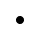Mobile3July 11, 2022

# The Introduction To Artificial Intelligence Algorithms #2 - TabuSearch

A small advance from the not so clever climbing algorithm to stand-alone software is the so-called TabuSearch algorithm. This algorithm is similar to a climbing algorithm, but contains one minor change.

The hill climbing algorithm (HillClimb) chooses the best one from various options and follows it. However, sometimes it can happen that we can "loop" when repeatedly choosing options / neighbors. We can imagine such a cycle, for example, by repeatedly getting to the same place in the labyrinth. Maybe we really need to back off a bit and look for another way.

For example, let's look at this labyrintt. At one point, we get into a cycle from which we can no longer get out. If we mark this intersection as forbidden, our algorithm will suddenly be able to find a solution

This algorithm therefore needs to store already visited solutions in order to avoid their repetition.

## Best thing since sliced bread

Now we could start making wise words ale but I don't like to do that. In this case, the details are irrelevant, and you probably won't need this algorithm in your life. But you can think about the questions yourself:

• How many items can I keep in the tab list?
• Do the items on this list have a time expiration date?
• Do I need to remember more than just the condition for my particular problem? (eg. number of steps to a given point, time, other parameters)

## Algorithm

So let's write our algorithm down:

1. Let's create one random solution s
2. Make a list of banned solutions tabu
3. Until we are satisfied with the solution s
4. neighbors of our solution s
5. we will remove all solutions from the neighbors that are in the tab list
6. s = best solution from neighbors
7. add s to tabu list

If we remove all lines with tab text from this algorithm, we get the climbing algorithm again 🙂

## Practical problem - 8 queens

This problem is my favorite problem for studying optimization algorithms. Its wording is as follows: "Arrange 8 queens on an 8x8 chessboard so that no pair of queens threatens each other." There are 92 such solutions, and we can get to the first solution relatively quickly by a simple brute-force method. One of these solutions is this:

Thus we can represent this solution as a vector (array) of size 8 with row index [5, 3, 1, 7, 4, 6, 0, 2] (the first column has a queen on the 5th row, the second on the 3rd)… However, I recommend solving this problem for at least 10 checkers on a 10x10 board. Then you will see real differences in the calculation speed. Let's denote this number as n

So let's try to find a solution using the tabu search algorithm:

1. Create a random solution (1) and a tabu list (2):

2. The termination condition (3) will be to find the first correct solution (we minimize the cost of the solution cost = number of collisions between queens):

3. We create n neighbors (3a), each of them is a copy of the original but the value on the i - th index we move its value by + 1

3b. We filter the neighbors according to the tabu list.

3c. And we will choose the best from them.

3d. Finally, we update the tabu list and we're done with the algorithm.

The entire functional program, including cost functions in the Kotlin language.

### Footnote

For a chessboard size of 10x10, I found a solution within 60 seconds using the bruteforce method. The TabuSearch method took about 1.5 - 3 seconds.

Try to think about why HillClimb is not the right choice for solving this problem and whether TabuSearch is also suitable for solving the 20x20 chessboard.

If you've guessed right that TabuSearch won't solve the 20x20 problem, next time we'll show you another method that can do it. Hooray for simulated annealing.